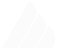# Mapping Arrays Like A Pro

Let's get started with some mapping. Previously I made a post about
filtering arrays like a pro and this one also will be somewhat same, so put your seat belts on.
In this post, I will show you some cool tricks and hacks to map arrays.

To map arrays like a PRO we will use Array.prototype.map() method.

## Mapping Number()

Using the Number object we can convert any string of arrays to numbers easily.

``````['1', '2', '-1', '0'].map(Number);
// -> [1, 2, -1, 0]``````

So, you might think that `Number.parseInt` will also work in this situation because it is also a function which we can pass into the map function, But no. See why:-

``````['1', '2', '-1', '0'].map(Number.parseInt);
// -> [1, NaN, -1, 0]``````

It results in [1, NaN, -1, 0] because we mostly use parseInt with one argument, But it takes two, input & radix.

``````/**
* @param {string} input
*/
parseInt('0011', 2)
// -> 3``````

Thus when we pass parseInt to Array.prototype.map, it passes 3 arguments to the parseInt, the element, index & the array.

``````/*  1st iteration (index is 0): */ parseInt("1", 0);  // 1
/*  2nd iteration (index is 1): */ parseInt("2", 1);  // NaN
/*  3rd iteration (index is 2): */ parseInt("-1", 2); // -1
/*  4th iteration (index is 2): */ parseInt("0", 2);  // -1``````

Checkout Mozilla Docs for better explaination of this

## Mapping Object()

Well, this one is a bit of useless 😂, By passing the `Object` into map you can get the primitive value of the element.

``````let arr = ['1', '2', '-1', [1,3], {a : 1}, 0, null]
arr.map(Object)
// -> [String{"1"}, String{"2"}, String{"-1"}, [1, 3], {a: 1}, Number{0}, {}]``````

You can also try this for fun.

``````Object.values(window).map(Object)
// OR
Object.values(window).map(String)
// OR
Object.values(window).map(Number)
// -> lot of things!``````

## Freezing Objects In An Array 🥶

By using the Object.freeze we can freeze all the objects inside the array.

``````let obj1 = { data: 'hello' };
let obj2 = { data: 'world' };
[obj1, obj2].map(Object.freeze)``````

## Mapping getOwnPropertyDescriptors()

Same as Object.freeze we can also pass Object.getOwnPropertyDescriptors to map all the propertyDescriptors.

``````[{a: 1}, {b: 2}].map(Object.getOwnPropertyDescriptors)
/* ->
[
{ a: { value: 1, writable: true, enumerable: true, configurable: true } },
{ b: { value: 2, writable: true, enumerable: true, configurable: true } }
]
*/``````

## Mapping CharCodes

Let's do some fun stuff :p
With the help of Array.fill() and String.fromCharCode method, we can map all the charCodes in an array.

``````new Array(100).fill(1).map(String.fromCharCode)
// -> This is soo weird that i can't even copy paste the output!``````

## Mapping Math

Let's also use map with the Math Object.

``````[0.2,1.5,-0.5,5,1,0,-0.8].map(Math.ceil)
// -> [1, 2, -0, 5, 1, 0, -0]
[0.2,1.5,-0.5,5,1,0,-0.8].map(Math.floor)
// -> [0, 1, -1, 5, 1, 0, -1]
[0.2,1.5,-0.5,5,1,0,-0.8].map(Math.abs)
// -> [0.2, 1.5, 0.5, 5, 1, 0, 0.8]``````

You can also try to map other Math methods.

## Mapping The Error Object

We can also directly map the Error Object.
Well, i don't know where will you find usefulness of this. 😂

``````['hello', 'world'].map(Error)
// -> [Error: hello at Array.map (<anonymous>) at <anonymous>:1:20, Error: world at Array.map (<anonymous>) at <anonymous>:1:20]``````

### Side Note

Same as my previous post about filtering arrays, you can also use those methods to map Boolean values into the array.

``````[undefined, null, 0, '', 'str', 2.5, 2, { a: 1 }, []].map(Boolean);
// -> [false, false, false, false, true, true, true, true, true]
[undefined, null, 0, 'str', 1, { a: 'b' }, [5, 6], [{ a: 5 }]].map(isArray)
// -> [false, false, false, false, false, false, true, true]``````

That's it for this post, I hope you enjoyed these mapping tricks, and i know most of them are not very useful, But sometimes fun is what all you need.

hope you like this, and now you are also a PRO at mapping arrays! 😉.

And Don't forget to comment down your favorite array tricks and tips and let me know your thought's about these tricks. See ya!.

And also don't forget to checkout my other post:- filtering arrays like a proMade with love and gatsby by me